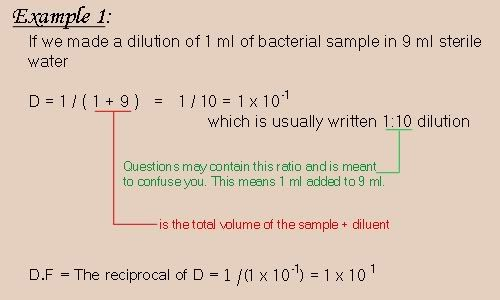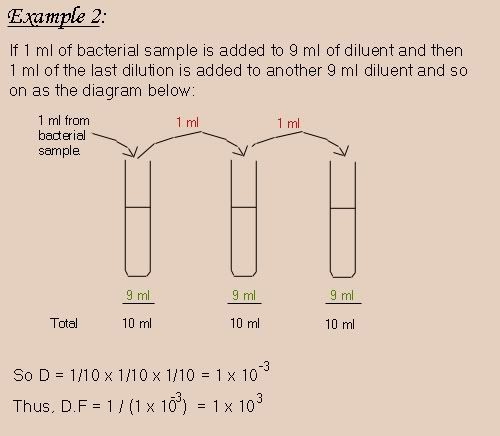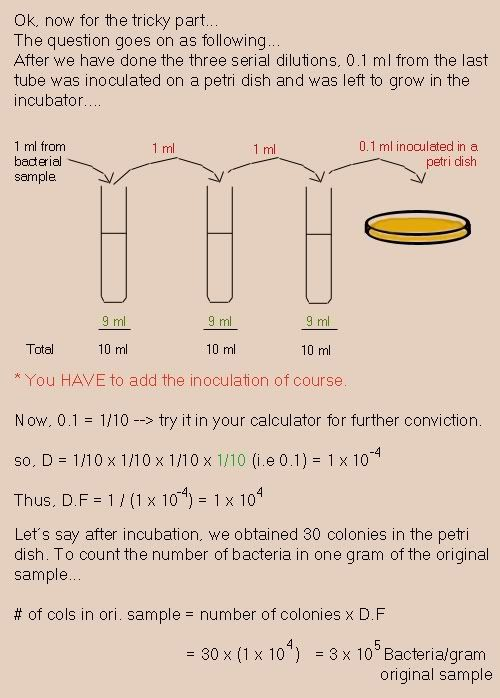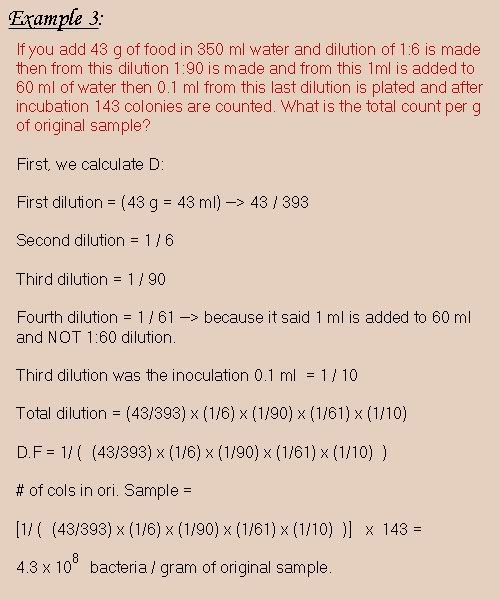# Diluting Ampicillin into LB Agar - (Mar/21/2009 )

Pages: 1 2 3 Next

Today was a very bad day at the university... beside that some students answered in the quiz question that if you get blue color in Gram staining that means your bacteria is Gram negativeI noticed most of the students including myself had trouble with the dilution process. So I thought maybe I'd make a quick explanation for what is dilution and how it is done and all the ratios and other confusing stuff. I tried my best to simplify it for people who find trouble in doing their homeworks, labwork, or other calculation problems regarding dilution questions and solutions.

So let's start out with definitions:

Dilution: is the mixing of a small accurately measured sample with a large volume of sterile water or normal saline called (diluents or dilution blank)

Laws:

Dilution = V of Sample / Total V of (sample + diluent)

Dilution Factor = Total V of (sample + diluent) / V of sample
** or we can simply say the reciprocal of Dilution

Starting off with this simple example to understand how these laws are applied.It has been known that if we use a larger volume we obtain a more accurate dilution.
So for better results, we use 1:1000 dilution. And that is by adding 1ml of sample to 999 ml of diluent. But practically we cannot use 999 ml of diluent. So we do what is called a serial dilution.

Serial Dilution: is a dilution made of a series of smaller dilution, and the total dilution is the product of each dilution in the series.

To understand this more, let's see this example.Here's an example combining all of the above.I hope this brief explanation proves helpful to you...let me know if i've made any mistakes...-Property of Yulia-

what makes me always confused is the last volume used for inoculation (0.1ml in your example)..

-strawberry-

Yeah that's why i almost jumped with 'HAVE' there :blush: sorry about that
i hope my mini-tutorial and examples helped you through it strawberry...-Property of Yulia-

I'm very thankful to you to provide the easier explaination...it's true that many undergrad students have problem in dilution..and the worst thing is if the lecturers/tutors assume that the students are familiar with the concept. it is true that they have learned it in high school, but most of the time they will forget..

Regarding the example 2 given, you dilute a sample (10 ml the final volume) 10x10x10=10 to the power of 3. and u inoculate 0.1 ml in your medium.so supposed the final volume of your medium is 1ml right?

-kent19-

This post has been promoted to an article

-bioforum-

Please explain me the conversion of % to ppm. I am damn confused.

-BHARGAVI-

BHARGAVI on Mar 23 2009, 03:09 AM said:

Please explain me the conversion of % to ppm. I am damn confused.

ppm means part per million. there fore x ppm = x parts of something to a million parts of diluent. % is something like "parts per hundred" so if its a 10% SDS solution. Its 10 g per hundres parts diluent which is dH20.

-MaggieRoara-

Thanks for nice explanation!!!

-Niraj-

Nice stuff!

Perhaps you can help me with this:

For real time pcr (detection of virus), one wants to measure the sensitivity of the assay by determining the minumun amount of viral copies per reaction that the assay is able to detect.
So, let´s say I have 8 ng/ul of target RNA in the sample (measured by nanospec). By using this formula:
Transcript length: 75nucleotides
Concentration: 8 ng/ul = 8 x 10-9 g/ul
Calculation: (8 x 10-9 g/ul / <75x 340>) x 6.022 x 10e23 =about 1,8e11 molecules per ul

I dilute this by factor 10-9 and I get 188 molecules per ul.

My PCR mix is 25ul that includes 2ul of this RNA sample. My assay is able to detect this dilution so can I say that the detection limit is 376 copies PER REACTION (I put 2 microliters and 2X 188/ul=376) or is the limit this 376 divided by the reaction volume of 25??

-Jka83-

Jka83 on Jul 21 2009, 12:14 PM said:

Nice stuff!

Perhaps you can help me with this:

For real time pcr (detection of virus), one wants to measure the sensitivity of the assay by determining the minumun amount of viral copies per reaction that the assay is able to detect.
So, let´s say I have 8 ng/ul of target RNA in the sample (measured by nanospec). By using this formula:
Transcript length: 75nucleotides
Concentration: 8 ng/ul = 8 x 10-9 g/ul
Calculation: (8 x 10-9 g/ul / <75x 340>) x 6.022 x 10e23 =about 1,8e11 molecules per ul

I dilute this by factor 10-9 and I get 188 molecules per ul.

My PCR mix is 25ul that includes 2ul of this RNA sample. My assay is able to detect this dilution so can I say that the detection limit is 376 copies PER REACTION (I put 2 microliters and 2X 188/ul=376) or is the limit this 376 divided by the reaction volume of 25??

Most likely a silly question, but where do you get the 340 , 6.022 and 10e23 from?-lyok-

bioforum on Mar 21 2009, 11:33 AM said:

<p>Today was a very bad day at the university... beside that some students answered in the quiz question that if you get blue color in Gram staining that means your bacteria is Gram negativeI noticed most of the students including myself had trouble with the dilution process. So I thought maybe I'd make a quick explanation for what is dilution and how it is done and all the ratios and other confusing stuff. I tried my best to simplify it for people who find trouble in doing their homeworks, labwork, or other calculation problems regarding dilution questions and solutions.

So let's start out with definitions:

Dilution: is the mixing of a small accurately measured sample with a large volume of sterile water or normal saline called (diluents or dilution blank)

Laws:

Dilution = V of Sample / Total V of (sample + diluent)

Dilution Factor = Total V of (sample + diluent) / V of sample
** or we can simply say the reciprocal of Dilution

Starting off with this simple example to understand how these laws are applied.It has been known that if we use a larger volume we obtain a more accurate dilution.
So for better results, we use 1:1000 dilution. And that is by adding 1ml of sample to 999 ml of diluent. But practically we cannot use 999 ml of diluent. So we do what is called a serial dilution.

Serial Dilution: is a dilution made of a series of smaller dilution, and the total dilution is the product of each dilution in the series.

To understand this more, let's see this example.Here's an example combining all of the above.I hope this brief explanation proves helpful to you...let me know if i've made any mistakes...</p> <div>-Property of Yulia-</div><hr>

</p> <div>-kent19-</div>

This does contain mistakes. In the description for example 3, the author shows that 43 g of food = 43 ml. This is only true if the density of the food = 1g/ml. Since we are not given the density of the food nor told that it is equal to that of pure water at 4°C, the calculation cannot be performed as described without undue assumptions by the author.

-Dr Teeth-

lyok on Jul 21 2009, 10:30 PM said:

Jka83 on Jul 21 2009, 12:14 PM said:

Nice stuff!

Perhaps you can help me with this:

For real time pcr (detection of virus), one wants to measure the sensitivity of the assay by determining the minumun amount of viral copies per reaction that the assay is able to detect.
So, let´s say I have 8 ng/ul of target RNA in the sample (measured by nanospec). By using this formula:
Transcript length: 75nucleotides
Concentration: 8 ng/ul = 8 x 10-9 g/ul
Calculation: (8 x 10-9 g/ul / <75x 340>) x 6.022 x 10e23 =about 1,8e11 molecules per ul

I dilute this by factor 10-9 and I get 188 molecules per ul.

My PCR mix is 25ul that includes 2ul of this RNA sample. My assay is able to detect this dilution so can I say that the detection limit is 376 copies PER REACTION (I put 2 microliters and 2X 188/ul=376) or is the limit this 376 divided by the reaction volume of 25??

Most likely a silly question, but where do you get the 340 , 6.022 and 10e23 from?

There are no silly questions.
The formula can be found from here:
http://www1.qiagen.com/resources/info/Guid...uantifying.aspx
"RNA standards"

-Jka83-

Ah I see, thanks for the link.

I do have a question: what do you mean with: I dilute this by factor 10-9 and I get 188 molecules per ul.

My PCR mix is 25ul that includes 2ul of this RNA sample. My assay is able to detect this dilution so can I say that the detection limit is 376 copies PER REACTION (I put 2 microliters and 2X 188/ul=376) or is the limit this 376 divided by the reaction volume of 25??

I do not understand your question.

In the end you simply have 376 molecules per 25µl and and you can detect this.

You can then say , I think , that you will detect 376 molecules per 25µl (=per reaction) or 376 molecules/25µl = X molecules per µl.

Is this not the same?

-lyok-

And for the lazy ones, like me